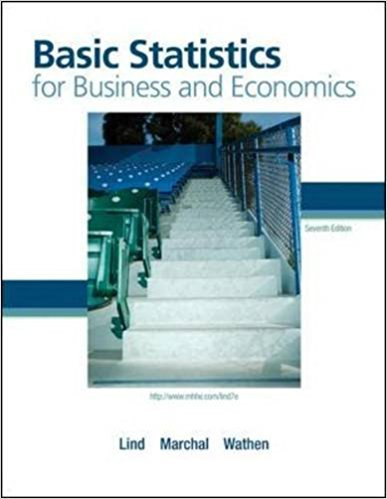×
Get Full Access to Basic Statistics For Business And Economics - 7 Edition - Chapter 6 - Problem 57e
Get Full Access to Basic Statistics For Business And Economics - 7 Edition - Chapter 6 - Problem 57e

×

# During the second round of the 1989 U.S. Open golfISBN: 9780077384470 64

## Solution for problem 57E Chapter 6

Basic Statistics for Business and Economics | 7th Edition

• Textbook Solutions
• 2901 Step-by-step solutions solved by professors and subject experts
• Get 24/7 help from StudySoup virtual teaching assistantsBasic Statistics for Business and Economics | 7th Edition

4 5 1 325 Reviews
31
4
Problem 57E

Problem 57E

During the second round of the 1989 U.S. Open golf tournament, four golfers scored a hole in one on the sixth hole. The odds of a professional golfer making a hole in one are estimated to be 3,708 to 1, so the probability is 1/3,709. There were 155 golfers participating in the second round that day. Estimate the probability that four golfers would score a hole in one on the sixth hole.

Step-by-Step Solution:
Step 1 of 3

Chapter 9 3/28/17 in-class notes Hypothesis Tests  Testing hypotheses: testing the validity of a claim/assumption about value of a population parameter (µ or p)  Null (H0) and Alternative (H a hypotheses  Two types of error in conclusion: Type I and Type II, with specific probabilities/risks  Hypothesis testing regarding the population mean (same as Ch 8) o Use the z distribution in known cases o Use the t distribution in unknown cases  Hypothesis testing regarding the population proportion o Always use the z distribution Hypothesis testing can be used to determine whether a statement/claim about the value of a population parameter (µ or p) should or should not be rejected based on x

Step 2 of 3

Step 3 of 3

##### ISBN: 9780077384470

Unlock Textbook Solution